# Accounting Ratios (Part - 1) Notes | Study TS Grewal Solutions - Class 12 Accountancy - Commerce

## Commerce: Accounting Ratios (Part - 1) Notes | Study TS Grewal Solutions - Class 12 Accountancy - Commerce

The document Accounting Ratios (Part - 1) Notes | Study TS Grewal Solutions - Class 12 Accountancy - Commerce is a part of the Commerce Course TS Grewal Solutions - Class 12 Accountancy.
All you need of Commerce at this link: Commerce

Page No. 4.91

Question:1
From the following compute Current Ratio: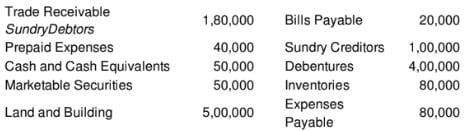Solution.
Current Assets = Trade Receivables + Pre-paid Expenses + Cash and Cash Equivalents + Marketable Securities + Inventories
= Rs 1,80,000 + Rs 40,000 + Rs 50,000 + 50,000 + 80,000 = Rs 4,00,000
Current Liabilities = Bills Payable + Sundry Creditors + Expenses Payable
= Rs 20,000 + Rs 1,00,000 + Rs 80,000 = Rs 2,00,000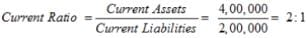Question:2
Calculate Current Ratio from the following information: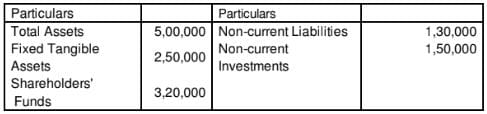Total Assets = Fixed Tangible Assets + Non - Current Investments + Current Assets 5,00,000 = 2,50,000 + 1,50,000 + Current Assets
Current Assets = 5,00,000 – 4,00,000 = Rs 1,00,000
Total Assets = Shareholder’s Funds + Non – Current Liabilities + Current Liabilities
5,00,000 = 3,20,000 + 1,30,000 + Current Liabilities
Current Liabilites = 5,00,000 - 4,50,000 = Rs 50,000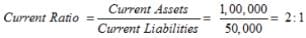Question:3
Current Ratio is 2.5, Working Capital is 1,50,000. Calculate the amount of Current Assets and Current Liabilities.
Solution: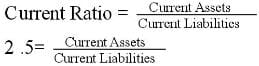Current Assets = 2 .5 Current Liabilities
Working Capital = Current Assets - Current Liabilities
1,50,000 = 2.5 Current Liabilities - Current Liabilities
Current Liabilities =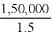Current Liabilities = Rs 1,00,000
Current Assets = 2 .5 Current Liabilities
Current Assets = 2.5 x 1,00,000 = Rs 2,50,000

Question:4
Working Capital is 9,00,000; Trade payables 90,000; and Other Current Liabilities are 2,10,000. Circulate Current Ratio.
Solution:
Working Capital = Rs 9,00,000
Current Liabilities = Trade Payables + Other Current Liabilities
= Rs 90,000 + Rs 2,10,000 = Rs 3,00,000
Working Capital = Current Assets – Current Liabilities
Rs 9,00,000 = Current Assets – Rs 3,00,000
Current Assets = Rs 9,00,000 + Rs 3,00,000 = Rs 12,00,000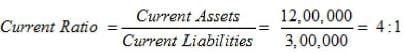Question:5
Working Capital 1,80,000; Total Debts 3,90,000; Long-Term Debts 3,00,000.
Calculate Current Ratio.

Solution:
Total Debts = 3,90,000
Long-term Debts = 3,00,000
Current Liabilities = Total Debts − Long-term Debts
= 3,90,000 − 3,00,000 = 90,000
Working Capital = Current Assets − Current Liabilities
1,80,000 = Current Assets − 90,000
Current Assets = 2,70,000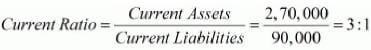Question:6
Current Assets are 7,50,000 and Working Capital is 2,50,000. Calculate Current Ratio.
Solution:

Current Assets = Rs 7,50,000
Working Capital = Rs 2,50,000
Working Capital = Current Assets – Current Liabilities
2,50,000 = 7,50,000 – Current Liabilities
Current Liabilities = 7,50,000 – 2,50,000 = Rs 5,00,000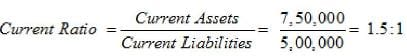Question:7
Trade Payables 50,000, Working Capital 9,00,000, Current Liabilities 3,00,000. Calculate Current Ratio.
Solution:

Working Capital = Current Assets - Current Liabilities
9,00,000 = Current Assets − 3,00,000
Current Assets = 9, 00, 000 + 3, 00, 000 = Rs 12,00,000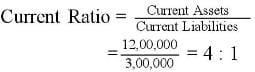Page No. 4.92

Question:8 A company had Current Assets of 4,50,000 and Current Liabilities of 2,00,000. Afterwards it purchased goods for 30,000 on credit. Calculate Current Ratio after the purchase.
Solution:

Current Assets = Rs 4,50,000
Current Liabilities = Rs 2,00,000
Purchase of Goods on Credit for Rs 30,000 will have two effects:
1. Increase Stock by Rs 30,000, Current Assets will thereby increase to Rs 4,80,000 Rs 4, 50, 000 + Rs30, 000
2. Increase Creditors by Rs 30,000 and therefore Current Liabilities will now be Rs 2,30,000 Rs2, 00, 000 + Rs30, 000

Question:9 Current Liablilites of a company were 1,75,000 and its Current Ratio was 2:1. It paid 30,000 to a Creditor. Calculate Current Ratio after payment.
Solution: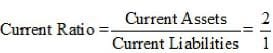Current Liabilities = Rs 1,75,000
Payment of Rs 30,000 to a Creditor will have two effects:
1. Decrease in Cash by Rs 30,000 and therefore Current Assets will decrease to Rs 3,20,000.
2. Decrease in Creditors by Rs 30,000 and this will decrease Current Liabilities to Rs 1,45,000.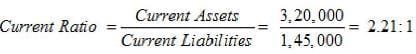Question:10
Ratio of Current Assets 3, 00, 000
to Current Liabilities 2, 00, 000

is 1.5:1. The accountant of the firm is interested in maintaing a Current Ratio of 2:1 by paying off a part of the Current Liabilities. Compute amount of the Current Liabilities that should be paid so that the Current Ratio at the level of 2:1 may be maintained.
Solution: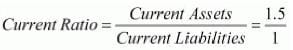The company is interested in maintaining the Current Ratio of 2:1 by paying off the liability.
Let the liability paid-off by the company = x
∴ New Current Assets = 3,00,000 − x
New Current Liabilities = 2,00,000 − x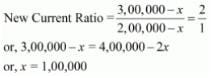Therefore, liability of Rs 1,00,000 need to be paid-off by the company in order to maintain the Current Ratio of 2 : 1.

Question:11
Ratio of Current Assets 8, 75, 000
to Current Liabilities 3, 50, 000 is 2.5:1. The firm wants to maintain Current Ratio of 2:1 by purchasing goods on credit. Compute amount of goods that should be purchased on credit.
Solution:
Current Assets = Rs 8,75,000
Current Liabilities = Rs 3,50,000
Current Ratio = 2.5:1
The business is interested to maintain its Current Ratio at 2:1 by purchasing goods on credit.
Let the amount of goods purchased on credit be ‘x’
Current Liabilities = Rs 3,50,000 + x
Current Assets = Rs 8,75,000 + x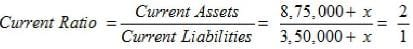8,75,000 + x = 7,00,000 + 2x
8,75,000 – 7,00,000 = 2x – x
1,75,000 = x
Therefore, goods worth Rs 1,75,000 must be purchased on credit to maintain the current ratio at 2:1.

Question:12
A firm had Current Assets of 5,00,000. It paid Current Liabilities of 1,00,000 and the Current Ratio became 2:1. Determine Current Liabilities and Working Capital before and after the payment was made.
Solution:

Firm disposed off liabilities of Rs 1,00,000 which results in decrease in current liabilities and current assets by the same amount.
After disposing liabilities:
Current Assets = Rs 4,00,000 Rs5, 00, 000– Rs1, 00, 000
And, Let Current Liabilities be x– Rs1,00,000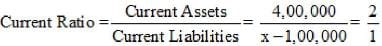4,00,000 = 2x – 2,00,000
6,00,000 = 2x
Therefore, x = 3,00,000
Current Liabilities after payment = x – Rs 1,00,000 = Rs 2,00,000
Working Capital after Payment = Current Assets – Current Liabilities
= Rs 4,00,000 – Rs 2,00,000 = Rs 2,00,000
Current Assets before payment = Rs 5,00,000
Current Liabilities before Payment = Rs 3,00,000
Therefore, Working Capital Before Payment = Current Assets – Current Liabilities
= Rs 5,00,000 – Rs 3,00,000 = Rs 2,00,000

Question:13
State giving reason, whether the Current Ratio will improve or decline or will have no effect in each of the following transactions if Current Ratio is 2:1:
(a)
(b)
Bills Payable discharged.
(c)
Bills Receivable endorsed to a creditor.
(d)
Payment of final Dividend already declared.
(e)
(f)
Bills Receivable endorsed to a Creditor dishonoured.
(g)
(h)
Sale of Fixed Assets Book Value of 50, 000 for 45,000.
(i)
Sale of FIxed Assets Book Value of 50, 000 for 60,000.
Solution:

Let’s assume Current Assets as Rs 2,00,000 and Current Liabilities as Rs 1,00,000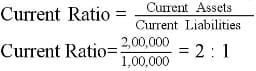(a) Cash paid to Trade Pay ables (say Rs 50,000)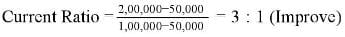(b) Bills Payable discharged (say Rs 50,000)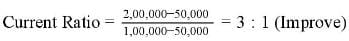(c) Bills Receivable endorsed to a creditor (say Rs 50,000)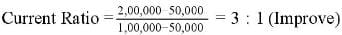(d) Payment of final Dividend already declared (say Rs 50,000)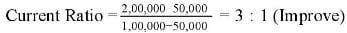(e) Purchase of Stock-in-Trade on credit (say Rs 50,000)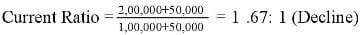(f) Bills Receivable endorsed to a Creditor dishonoured (say Rs 50,000)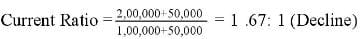(g) Purchase of Stock-in-Trade for cash (say Rs 50,000)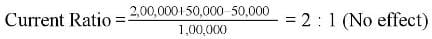(h) Sale of Fixed Assets Bookvalue of Rs50, 000 for Rs 45,000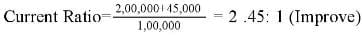(i) Sale of Fixed Assets Book value of Rs50, 000 for Rs 60,000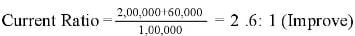Question:14
State giving reasons, which of the following transactions would improve, reduce or not change the Current Ratio, if Current Ratio of a company is (i)
1:1; or (ii) 0.8:1:
(a)

(b)
(c)
(d)
Payment of Dividend payable.
(e)
Bills Payable discharged.
(f)
Bills Receivable endorsed to a Creditor.
(g)
Bills Receivable endorsed to a Creditor dishonoured.
Solution:
(i) Let’s assume Current Assets as Rs 1,00,000 and Current Liabilities as Rs 1,00,000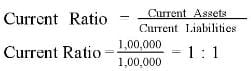(a) Cash paid to Trade Payables (say Rs 50,000)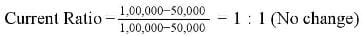(b) Purchase of Stock-in-Trade on credit (say Rs 50,000)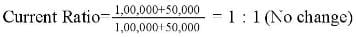(c) Purchase of Stock-in-Trade for cash (say Rs 50,000)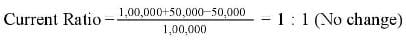(d) Payment of Dividend (say Rs 50,000)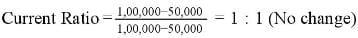(e) Bills Payable discharged (say Rs 50,000)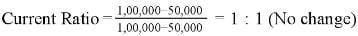(f) Bills Receivable endorsed to a Creditor (say Rs 50,000)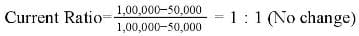(g) Bills Receivable endorsed to a Creditor dishonoured (say Rs 50,000)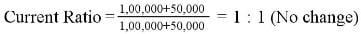(ii) Let’s assume Current Assets as Rs 80,000 and Current Liabilities as Rs 1,00,000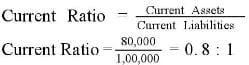(a) Cash paid to Trade Payables (say Rs 50,000)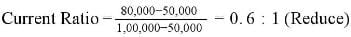(b) Purchase of Stock-in-Trade on credit  (say Rs 50,000)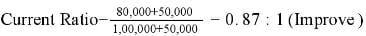(c) Purchase of Stock-in-Trade for cash (say Rs 50,000)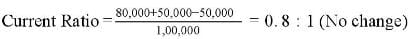(d) Payment of Dividend (say Rs 50,000)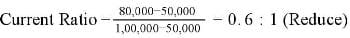(e) Bills Payable discharged (say Rs 50,000)(f) Bills Receivable endorsed to a Creditor (say Rs 50,000)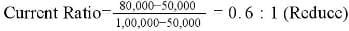(g) Bills Receivable endorsed to a Creditor dishonoured (say Rs 50,000)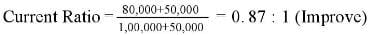Page No. 4.93

Question:15
From the following information, calculate Liquid Ratio: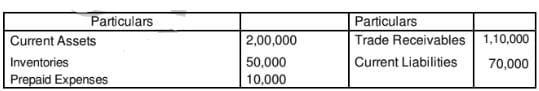Solution:
Quick Assets or Liquid Assets = Currents Assets – Inventories – Pre-paid Expenses
= Rs 2,00,000 – Rs 50,000 – Rs 10,000 = Rs 1,40,000
Current Liabilities = Rs 70,000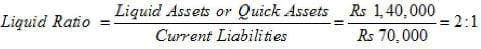Question:16
Quick Assets 1,50,000; Inventory Stock

40,000; Prepaid Expenses 10,000; Working Capital 1,20,000. Calculate Current Ratio.
Solution:

Quick Assets = 1,50,000
Inventory = 40,000
Prepaid Expenses = 10,000
Current Assets = Quick Assets + Inventory + Prepaid Expenses
= 1,50,000 + 40,000 + 10,000 = 2,00,000
Working Capital = Current Assets − Current Liabilities
1,20,000 = 2,00,000 − Current Liabilities
Current Liabilities = 80,000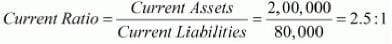Question:17 Current Assets 3,00,000; Inventories 60,000; Working Capital 2,52,000. Calculate Quick Ratio.
Solution:
Current Liabilities = Current Assets − Working Capital
= 3,00,000 − 2,52,000 = 48,000
Quick Assets = Current Assets − Stock
= 3,00,000 − 60,000 = 2,40,000

Question:18
Working Capital 3,60,000; Total :Debts 7,80,000; Long-term Debts 6,00,000; Inventories 1,80,000. Calcltate Liquid Ratio.
Solution:

Current Liabilities = Total Debts − Long-term Debts
= 7,80,000 − 6,00,000 = 1,80,000
Current Assets = Current Liabilities + Working Capital
= 1,80,000 + 3,60,000 = 5,40,000
Quick Assets = Current Assets − Stock
= 5,40,000 − 1,80,000 = 3,60,000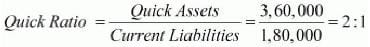Question:19
Current Liabilities of a company are 6,00,000. Its Current Ratio is 3 : 1 and Liquid Ratio is 1 : 1. Calculate value of Inventory
Solution: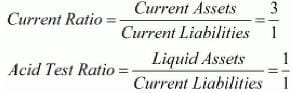Current Liabilities = 6,00,000
Current Assets = 3 × Current Liabilities
= 3 × 6,00,000 = 18,00,000
Liquid Assets = 1 × 6,00,000 = 6,00,000
Inventory = Current Assets − Liquid Assets
= 18,00,000 − 6,00,000 = 12,00,000

Question:20
X Ltd. has a Current Ratio of 3.5 : 1 and Quick Ratio of 2 : 1. If the Inventories is 24,000; calculate total Current Liabilities and Current Assets.
Solution: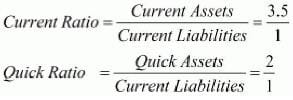Let Current Liabilities be = x
Current Assets = 3.5 x
Quick Assets = 2 x
Stock = Current Assets − Quick Assets
24,000 = 3.5 x − 2 x
or, 24,000 = 1.5 x
x = 16,000
Current Liabilities = x = Rs 16,000
Current Assets = 3.5 x = 3.5 × 16,000 = Rs 56,000

Question:21
X Ltd. has Current Ratio of 4.5 : 1 and a Quick Ratio of 3 : 1. If its inventory is 36,000, find out its total Current Assets and total Current Liabilities.
Solution: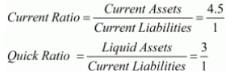Inventory = 36,000
Let Current Liabilities be = x
Current Assets = 4.5x
Quick Assets = 3x
Stock = Current Assets − Quick Assets
36,000 = 4.5x − 3x
x = 24,000
Current Assets = 4.5x = 4.5 × 24,000 = 1,08,000
Liquid Assets= 3x = 3 × 24,000 = 72,000

Question:22
Current Ratio 4; Liquid Ratio 2.5; Inventory 6,00,000. Calculate Current Liabilities, Current Assets and Liquid Assets.
Solution: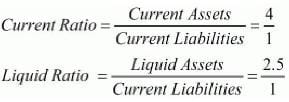Inventory = 6,00,000
Let Current Liabilities be = x
Current Assets = 4x
Quick Assets = 2.5x
Stock = Current Assets − Quick Assets
6,00,000 = 4x − 2.5x
x = 4,00,000
Current Assets = 4x = 4 × 4,00,000 = 16,00,000
Liquid Assets = 2.5x = 2. 5× 4,00,000 = 10,00,000

Question:23
Current Liabilities of a company are 1,50,000. Its Current Ratio is 3 : 1 and Acid Test Ratio Liquid Ratio is 1 : 1. Calculate values of Current Assets, Liquid Assets and Inventory.
Solution: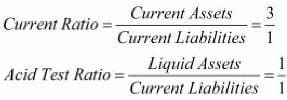Current Liabilities = 1,50,000
Current Assets = 3 × Current Liabilities
= 3 × 1,50,000 = 4,50,000
Liquid Assets = 1 × 1,50,000 = 1,50,000
Inventory = Current Assets − Liquid Assets
= 4,50,000 − 1,50,000 = 3,00,000

Question:24 Xolo Ltd.'s Liquidity Ratio is 2.5 : 1. Inventory is 6,00,000. Current Ratio is 4 : 1. Find out the Current Liabilities.
Solution: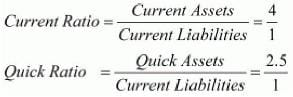Let the Current Liabilities be = x
Current Assets = 4x
Quick Assets = 2.5x
Stock = Current Assets − Quick Assets
6,00,000 = 4x − 2.5x
or, x = 4,00,000
Current Liabilities = x = Rs 4,00,000

Question:25
Current Assets of a company is are 5,00,000. Its Current Ratio is 2.5 : 1 and Quick Ratio is 1 : 1. Calculate value of Current Liabilities, Liquid Assets and Inventory.

Solution: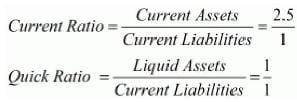Current Assets = 5,00,000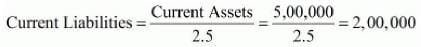Liquid Assets = Current Liabilities × 1 = 2,00,000
Inventory = Current Assets − Quick Assets
= 5,00,000 − 2,00,000 = 3,00,000

The document Accounting Ratios (Part - 1) Notes | Study TS Grewal Solutions - Class 12 Accountancy - Commerce is a part of the Commerce Course TS Grewal Solutions - Class 12 Accountancy.
All you need of Commerce at this link: CommerceUse Code STAYHOME200 and get INR 200 additional OFF

## TS Grewal Solutions - Class 12 Accountancy

52 docs

Track your progress, build streaks, highlight & save important lessons and more!

,

,

,

,

,

,

,

,

,

,

,

,

,

,

,

,

,

,

,

,

,

;